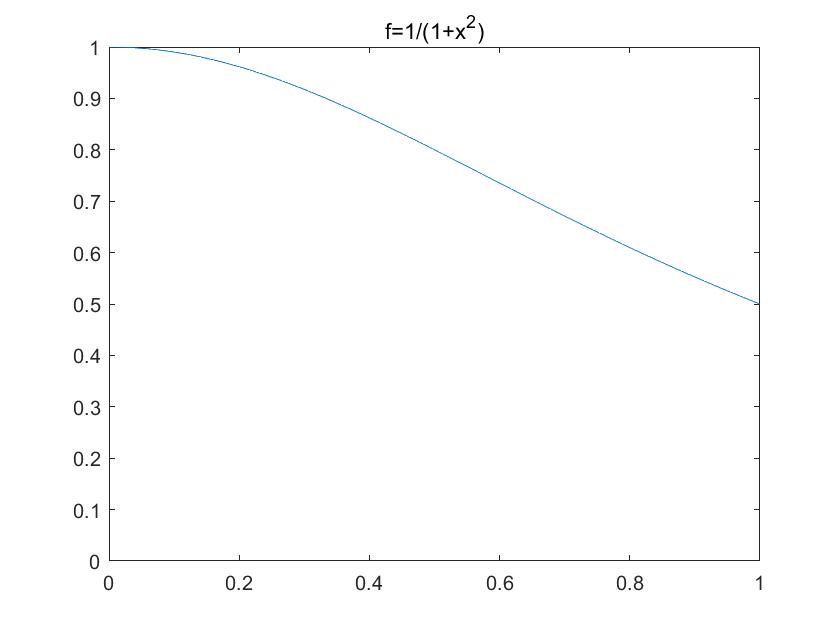# 如何用带权重的蒙特卡洛计算积分？

### 数值积分

$$\int_0^1 \frac{1}{1+x^2}=\arctan x|_0^1=\frac{\pi}{4}\approx{0.7854}$$

### 均匀取点

%matlab code
clear;
x=linspace(0,1,200); %平均分成200个样本
y=1./(1+x.^2);
int=mean(y) %结果为0.7852

### 均匀分布的蒙特卡洛取点

%matlab code
clear;
x=rand(1,200);%产生(0,1)区间均匀分布的200个随机数
y=1./(1+x.^2);
int2=mean(y)  %结果介于0.76~0.80$$w=\frac{4}{3}(2-x)$$

%matlab code
clear;
x=rand(1,200);
w=2-sqrt(4-3*x);
I=1./(1+w.^2)./(4-2*w)*3;
int3=mean(I)  %结果介于0.783~0.787